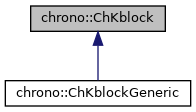chrono::ChKblock Class Referenceabstract

Description

Base class for representing items which introduce block-sparse matrices, that is blocks that connect some 'variables' and build a matrix K in a sparse variational inequality VI(Z*x-d,K):

Note that K blocks often have a physical interpretation as stiffness, but not always, for example they can represent hessians. Note that all blocks in K, all masses and constraint jacobians Cq are not really assembled in large matrices, so to exploit sparsity.

#include <ChKblock.h>

Inheritance diagram for chrono::ChKblock:[legend]

Public Member Functions

virtual size_t GetNvars () const =0
Returns the number of referenced ChVariables items.

virtual ChMatrixRef Get_K ()=0
Access the K stiffness matrix as a single block, referring only to the referenced ChVariable objects.

virtual void MultiplyAndAdd (ChVectorRef result, ChVectorConstRef vect) const =0
Computes the product of the corresponding blocks in the system matrix (ie. More...

Add the diagonal of the stiffness matrix block(s) as a column vector to 'result'. More...

virtual void Build_K (ChSparseMatrix &storage, bool add=true)=0
Writes (and adds) the K matrix associated to these variables into a global 'storage' matrix, at the offsets of variables. More...

◆ Build_K()

 virtual void chrono::ChKblock::Build_K ( ChSparseMatrix & storage, bool add = true )
pure virtual

Writes (and adds) the K matrix associated to these variables into a global 'storage' matrix, at the offsets of variables.

Most solvers do not need this: the sparse 'storage' matrix is used for testing, for direct solvers, for dumping full matrix to Matlab for checks, etc.

Implemented in chrono::ChKblockGeneric.

 virtual void chrono::ChKblock::DiagonalAdd ( ChVectorRef result )
pure virtual

Add the diagonal of the stiffness matrix block(s) as a column vector to 'result'.

NOTE: 'result' must already have the size of the total variables & constraints in the system.

Implemented in chrono::ChKblockGeneric.